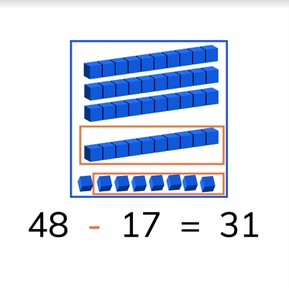Subtraction to 50 with subtrahends >10

# Subtraction to 50 with subtrahends >10

I can subtract numbers greater than 10 from numbers to 50.8,000 schools use Gynzy92,000 teachers use Gynzy1,600,000 students use Gynzy

## General

Students learn to subtract numbers greater than 10 from numbers to 50 without regrouping tens.

2.NBT.B.5

## Relevance

It is important that students can do subtraction to 50, so they can determine how many they have left.

## Introduction

Start by practicing on a number line to 50 by asking students to drag the numbers to where they belong on the number line. Then do a subtraction problem on the number line to 30 with a minuend of greater than 10.

## Development

Start by discussing the importance of subtraction to 50. This learning goal is explained in three different methods, visually, in the abstract, and in story form. You can use the blue menu in the bottom right to select the method that is best suited to your classroom needs. Otherwise start at the first which is the visual method. Explain how to subtract using the blocks on the interactive whiteboard. Show them the total number of blocks (blue) and then how many are taken away (orange). Discuss how many you have left. Then have students practice a set of visual subtraction problems on their own. Next, explain how to subtract in the abstract, so without visual support. Show students how they can jump on a number line to solve these subtraction problems. Show students that they can first subtract the tens and then the ones from the starting number(minuend). Show students two other methods of subtraction other than the number line. Emphasize that they can choose the method that works best for them. Then have students practice a set of abstract subtraction problems. Finally discuss the steps required to solve a story problem and do a story problem as s class. Then ask students to solve a story problem in pairs. Each method has explanation by the teacher, practice problems to do as a class, and then practice problems for the students to do to check their understanding.

Check that students are able to subtract numbers to 10 from numbers to 50 by asking the following questions:
- Why is it useful to be able to subtract to 50?
- What does the first number in a subtraction problem tell us?

## Guided practice

Students are first given a subtraction problem with visual support, then an abstract problem, and are then given a story problem to solve.

## Closing

As a class, do a visual, abstract, and story subtraction problem.

## Teaching tips

Students who have difficulty with this goal can be supported by making use of manipulatives, like blocks, to use when solving the subtraction problems.

## Instruction materials

Optional: blocks or other manipulatives

### The online teaching platform for interactive whiteboards and displays in schools

• Save time building lessons

• Manage the classroom more efficiently

• Increase student engagement